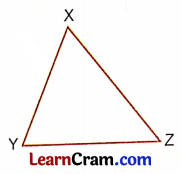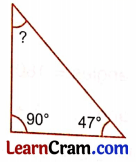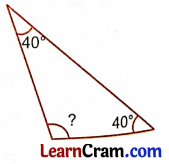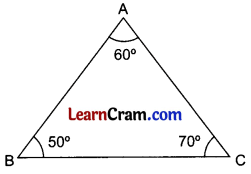# DAV Class 5 Maths Chapter 16 Worksheet 2 Solutions

The DAV Class 5 Maths Solutions and DAV Class 5 Maths Chapter 16 Worksheet 2 Solutions of Triangles offer comprehensive answers to textbook questions.

## DAV Class 5 Maths Ch 16 Worksheet 2 Solutions

Question 1.
Measure the angles of the triangle given below. What is the sum of the three angles?Solution:
∠X = 50°
∠Y = 60°
∠Z = 70°
∠X + ∠Y + ∠Z = 50° + 60° + 70° = 180°Question 2.
Find which of the following can be the measures of three angles of a triangle.
(a) 35°, 80°, 65°
Solution:
35°+ 80°+ 65° = 180°
Yes (The sum of angles of the triangle is 180°)

(b) 15°, 75°, 90°
Solution:
15° + 75° + 90° = 180°
Yes (The sum of angles of the triangle is 180°)

(c) 70°, 70°, 70°
Solution:
70° + 70° + 70° = 210°
No (The sum of the angles of the triangle is 180°)

(d) 45°, 59°, 81°
Solution:
45° + 59° + 81° = 185°
No (The sum of angles of a triangle is 180°)

Question 3.
Can you draw a triangle with two right angles? Give reasons.
Solution:
No, because the sum of 3 angles of a triangle will not be equal to 180°.

Question 4.
Can you draw a triangle with two obtuse angles? Give reasons.
Solution:
No, because the sum of 3 angles will exceed 180° so drawing a triangle is not possible with two obtuse angles.Question 5.
Find the missing angle in each of the following:
(a)Solution:
The sum of 3 angles of the triangle is 180°
So let the missing angle be x°.
x° + 65° + 40° = 180°
⇒ x° + 105° = 180°
⇒ x° = 180° – 105°
⇒ x° = 75°

(b)Solution:
The sum of 3 angles of the triangle is 180°
Let the missing angle be x°.
x° + 90° + 47° = 180°
⇒ x° + 137° = 180°
⇒ x° = 180° – 137°
⇒ x° = 43°

(c)Solution:
Let the missing angle be x°
40° + x° + 40° = 180° (Sum of angles of triangle is 180°)
⇒ 80° + x° = 180°
⇒ x° = 180° – 80°
⇒ x° = 100°

Question 6.
The sum of two angles of a triangle is 110°. Find the measure of the third angle.
Solution:
Let the third angle be x°.
Sum of two angles + third angle = 180°
⇒ 110° + x° = 180°
⇒ x° = 180° – 110°
⇒ x° = 70°

Question 7.
Two angles of a triangle are 100° and 55° respectively. Find the third angle.
Solution:
Two angles are 100° and 55°.
Let the third angle be x°.
So 100° + 55° + x° = 180° (sum of angle of triangle is 180°)
⇒ 155° + x° = 180°
⇒ x° = 180° – 155°
⇒ x° = 25°DAV Class 5 Maths Chapter 16 Worksheet 2 Notes

Properties of a Triangle

Property I:
The sum of the measures of the three angles of a triangle is always 180°.
Explanation:∠A + ∠B + ∠C = 60° + 50° + 70° = 180°

Example 1.
Find out the third angle of a triangle when the other two angles are given.
∠A = 55°
∠B = 30°
Find the ∠C =?
Solution:
Sum of angles = 180°
So, ∠A + ∠B + ∠C = 180°
⇒ 55° + 30° + ∠C = 180°
⇒ 85° + ∠C = 180°
⇒ ∠C = 180° – 85° = 95°
So the third angle is 95°.

Property II:
The sum of the lengths of any two sides of a triangle is greater than the length of the third side.
Explanation:AB = 4 cm
AC = 3 cm
BC = 5 cm
AB + BC = 9 cm
9 is greater than 3 or BC + AC = 8 cm
AB = 4 cm
8 is greater than 4 or AB + AC = 7 cm
BC = 5 cm
7 is greater than 5
So we observe that the sum of any two sides of a triangle is always greater than the third side.Example 2.
Can we draw a triangle with line segments of lengths 2 cm, 3 cm, and 8 cm?
Solution:
Length of first side = 2 cm
Length of second side = 3 cm
Length of third side = 8 cm
Sum of first and second side = 2 cm + 3 cm = 5 cm
Length of third side = 8 cm
8 > 5 or 5 < 8
So, we cannot draw a triangle with the line segments.